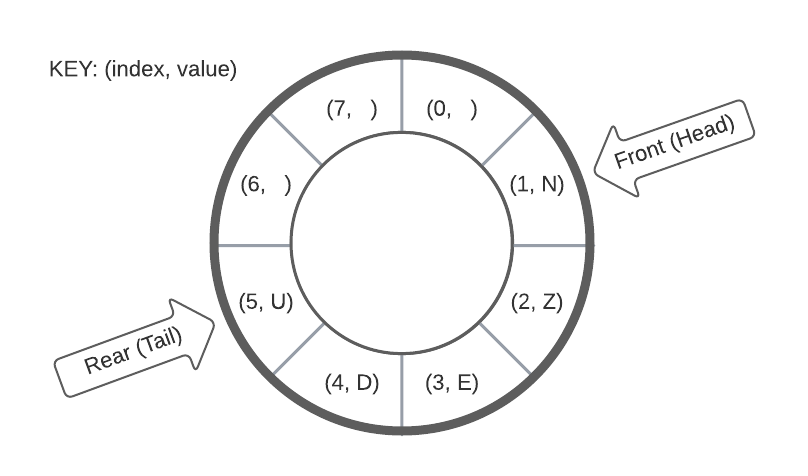Login or Create an Account to view the mark scheme, comment, and add to a test
(a).
Consider the array implementation of a circular queue illustrated below. Each element in the queue is characterised by both an index and value, indicating the underlying array index and element value respectively.(i).
By manipulating the circular queue represented above, draw the result of enqueuing letters "C", "A" & "T" (in their provided written order from left to right i.e. x3 separate enqueue operations) followed by one dequeue operation.

(ii).
Given an array implementation of the circular queue above, state the condition upon which it would be identified as FULL.

(b).
Describe the key functionality of a circular queue.

(c).
Compare and contrast circular queues with linear queues.


HomeThe Right Test - Fast